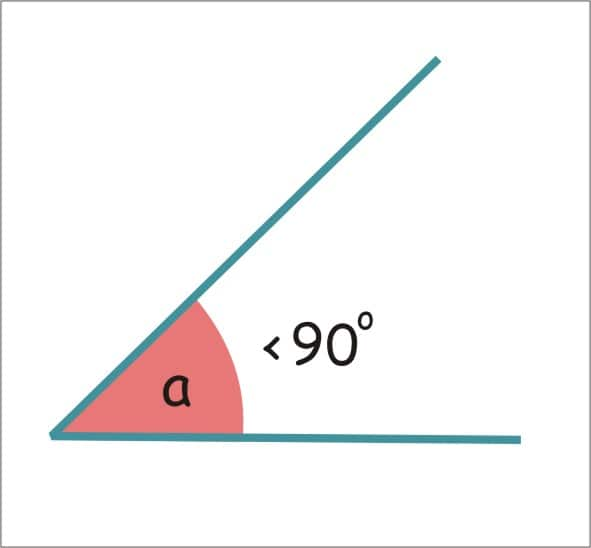# Branches of Mathematics

## What are the Branches of Mathematics?

Introduction:

Mathematics:

Mathematics is the theoretical science of quantity, number, and space, either as abstract concepts (pure mathematics) or as applied to other disciplines (applied mathematics).

It is the science of structure, order, and relation that has evolved from elemental practices of counting, measuring, and describing the shapes of objects. This basically deals with logical reasoning and quantitative calculation and its development have involved in increasing degree if idealization of its subject matter.

Branches of mathematics:

Mathematics has become vaster over the years. Mathematics is broadly divided into pure mathematics and applied mathematics. Applied mathematics is which can be applied to real world problems.
There are many branches of mathematics namely Algebra, Geometry, Arithmetic, Trigonometry, calculus etc.

Algebra:

Algebra is from the Arabic word al-jabr which means a reunion of broken parts. It is one of the broad parts of mathematics. In general term, algebra is the study of mathematical symbols and the rules for manipulating these symbols. It is the unifying thread of all the mathematics. It includes everything from solving the study of abstractions to solving the elementary equations.

The most basic part is the elementary algebra and the most abstract part is the modern or abstract algebra. Elementary algebra is considered to be essential for any study of mathematics, science or engineering as well as in economics and medicine. Likewise, abstract algebra is a major area in advances mathematics, studied mainly by professional mathematicians.

Formulas of algebra:
• • a²-b²=(a-b)(a+b)

• • (a+b)²=a²+2ab+b²

• • a²+b²=(a-b)²+2ab

• • (a-b)²=a²-2ab+b²

• • (a+b+c)²=a²+b²+c²-2ab-2ac+2bc

• • (a+b)³=a³+3a²b+3ab²+b³ ; (a+b)³=a³+b³+3ab(a+b)

• • (a-b)³=a³-3a²b+3ab²-b³

• • a³-b³=(a-b)(a²-ab+b²)

• For example:
Find out 5²-3²
Using the formula:
a²-b²=(a-b)(a+b)
Where a=5 and b=3
(a-b) (a+b)
(5-3) (5+3)
2×8 = 16

Arithmetic:

Arithmetic is one of the branches of maths that composed of the properties of the application on addition, subtraction, multiplication, and division, and also the study of numbers. It is a part of elementary number theory.

The fundamental arithmetic procedures include addition, multiplication, subtraction, and division also these add more advanced operations such as square roots, exponentiation, logarithmic functions, percentages, and also trigonometric functions

• It is the basic sign in the four operations of arithmetic. The addition of two whole numbers is the total amount of those sums combined. It is represented by a plus sign “+”. For example 5+2=7 i.e. 5 add 2 are equal to 7.
The Addition has several important properties. It is commutative. Commutative means the order does not matter.

Commutative property of addition:

3+8=8+3
And the other is Associative. Associative means when one adds more than two numbers, the order in which the addition is performed does not matter.

Associative property of addition:

2+3+4=2+3+4
If (2+3) +4=2+ (3+4)
If the addition is done for the number in the braces i.e. (2+3) and (3+4)
The answer will be the same 5+4=9 and 2+7=9.

Identity element:

It is that when adding any number to zero the quantity does not change. It is also known as additive identity. For example 5+0=5
Likewise a+0=0+a=a

Successor and predecessor:

The successor and predecessor refer to the numbers directly after or directly before a given number. For example, if the given number is 18. The successor number is 19 and the predecessor number is 17.

The items/ terms predecessor and successor apply only to the whole numbers and not any fractions, decimals or negative numbers. Every whole number has a successor.

• Subtraction:

• Subtraction is also an arithmetic operation next to addition. It represents the operation of removing objects from a collection. The result of subtraction is called the difference. It is represented by a minus sign “-“

For example 5-2=3

It represents decreasing abstracts and physical quantities using different kinds of objects including negative numbers, fractions, vectors, decimal, matrices etc.

It follows the important pattern. Subtraction is anti-commutative. It means that if one reverses the terms in a difference left-to-right then the result is the negative of the original result.

For example a-b=-(b-a) if a=4 and b=2
Then a-b- 4-2=2
-(b-a) =(2-4)= -2

Non-associativity:

Subtraction is non-associative. When one tries to define repeated subtraction the expression is such that a-b-c but it should be defined as (a-b)-c or a-(b-c). The two possibilities give two different answers.

• Multiplication:

• Multiplication is one of the four elementary operations of arithmetic. The multiplication symbol is represented by cross “×”.
The multiplication of whole numbers may be considered as repeated addition, that is the multiplication of two numbers is equivalent to adding as many copies of one of them, the multiplicand, as the value of the other, the multiplier. The multiplier can be written first and the multiplicand the second, both can be called factors.

For example 3×4=4+4+4=12
Here the factors of 3×4 = 12.

Commutative property of multiplication:

The order in which two numbers are multiplied is not taken into consideration.
For example: x.y= y.x

Associative property:

Expressions solely involving multiplication or addition are invariant with respect to the order of operations.
(x.y).z = x. (y.z)

Distributive property:

It holds with respect to multiplication over addition.
x. (y+z) = x.y + x.z

Identity element:

The multiplicative identity is 1. Anything multiplied by 1 is itself.
x.1=1

Property of 0:

Any number multiplies by 0 is 0.
x.0 =0

Rules of multiplication:
 Types of numbers Operation Result Example Positive ×positive Multiply Positive 1×5=5 Negative ×negative Multiply Positive (-1)×(-5)= 5 Positive ×negative Multiply Negative 1×(-5)=-5 Negative ×negative Multiply Negative (-1)×5=-5

• Division:

• The Division is one of the elementary operations in arithmetic like the other three operations. The Division is the distribution of numbers. Dividing integers is the opposite operation of multiplication. The rules for division of integers/figure are the same as multiplication.

The division symbol is represented by “÷”

For Example a÷b or a/b
The division formula is- dividend ÷ divisor = quotient or
Dividend/divisor = quotient

The dividend is the number to be divided. The Divisor is the number to be divided with quotient and the result to be found after division.

For example:
30÷1= 30
221÷31
13)221(17.
Therefore the result is 17.

Geometry:

A branch of mathematics concerned with size, shape, properties of space, and relative position of figures is called Geometry. A mathematician who undertakes the work in the field of geometry is called a geometer.
Geometry is derived from two Latin words, geo + metron meaning earth and measurement. The geometry is studied to find the length, area, volume of a different plane and solid figures.

• Point:

• A point is a precise location in a place or a plane. It is represented by a dot. Point is not a thing but a place. It should be noted that the point has no dimension and rather it has the only position.
Line: It is straight having no thickness and extends in both directions without end.
Line segment: if a line has a starting and end points then it is called a line segment.
Ray: If a line has a starting point and no end point it is called a ray.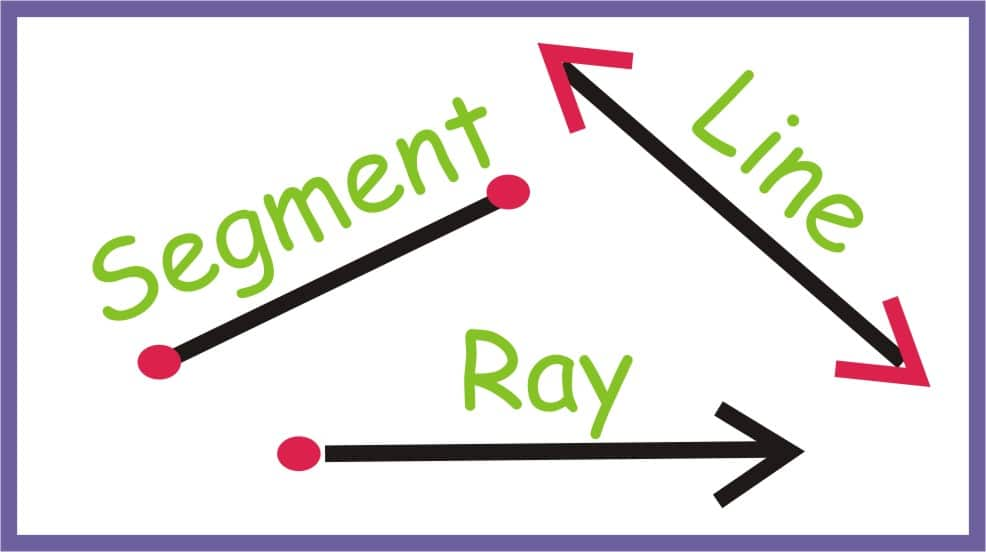• Angles:

• The angles are of different types and they are as follows:

Acute angle:

Acute angle means sharp is an angle smaller than a right angle. I.e. it can range between 0-90 degrees.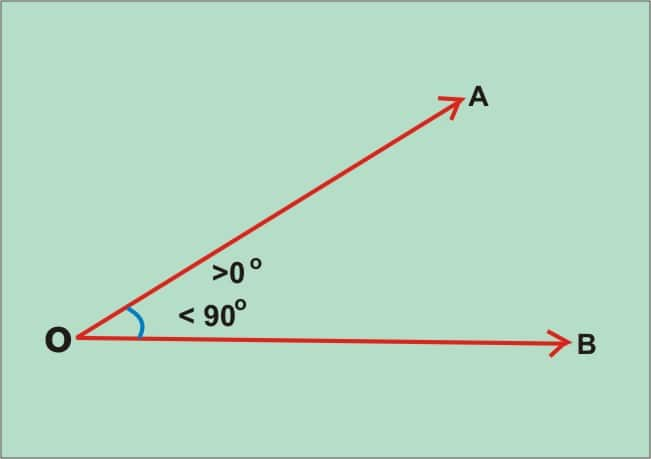Obtuse angle:

It is an angle more than 90 degrees but less than 180 degrees.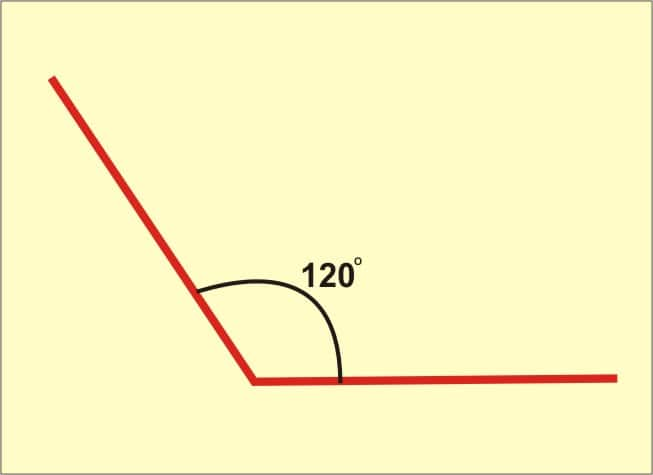Right angle:

It is an angle of 90 degrees.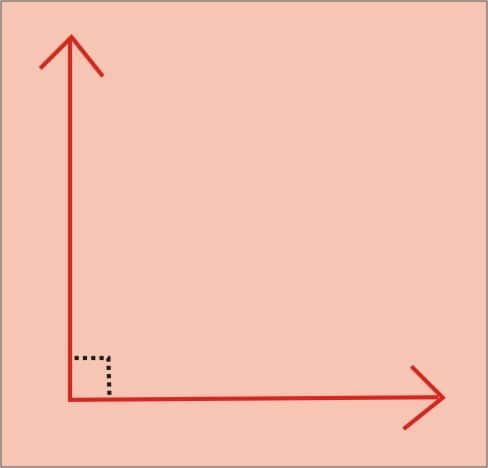Straight angle:

It is an angle of 180 degrees. Such an angle is formed by a straight line.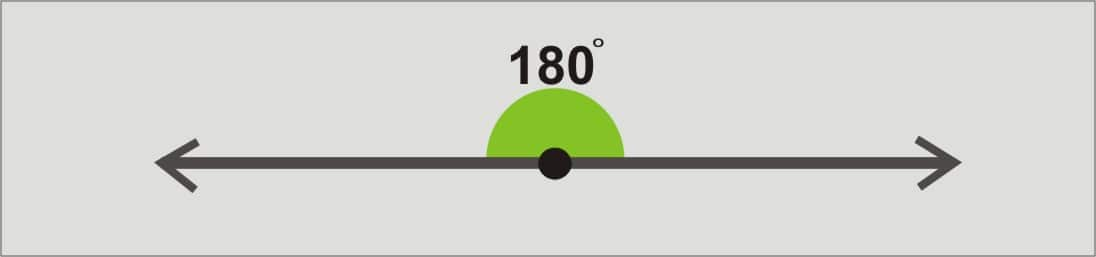Polygon:

Polygon is a figure that is bounded by a finite chain of straight line segment closing in a loop to form a closed polygonal chain or circuit.

The name poly refers to multiple. An n-gon is a polygon with n sides. Example: a triangle is a 3-gon polygon.

Different types of the polygon:

Triangle:

It is a three-sided polygon whose sum of internal angle always sums to 180 degrees. There are types like the equilateral triangle – has 3 equal sides, isosceles triangle- has 2 equal sides and angles, scalene triangle- has all the 3 unequal sides and angles.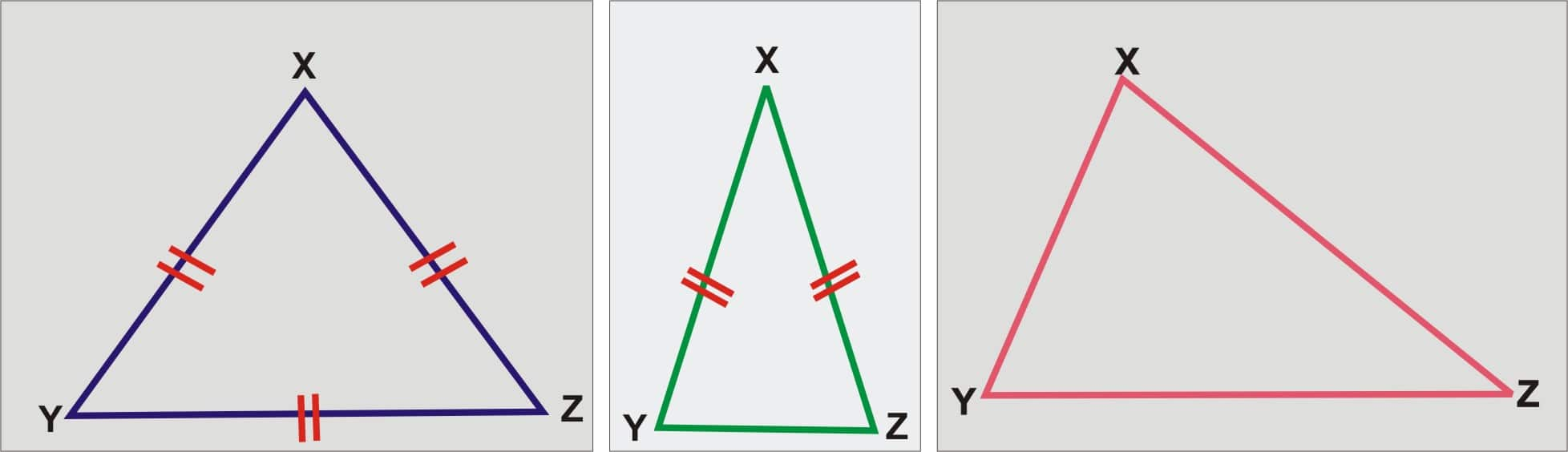It is a four-sided polygon with four edges and vertices. The sum of internal angles is 360 degrees. The types are square, rectangle, parallelogram, rhombus, and trapezium.
Square has all the four sides equal which are at right angles.
In a Rectangle, opposite sides are equal and all angles are right angled.
The Parallelogram has two pairs of parallel sides. In measurement the opposite sides and angles are equal.
In a Rhombus, all the four sides to be of equal in length. The internal angle is of not 90 degrees.
In a Trapezium has one pair of opposite sides are said to be equal.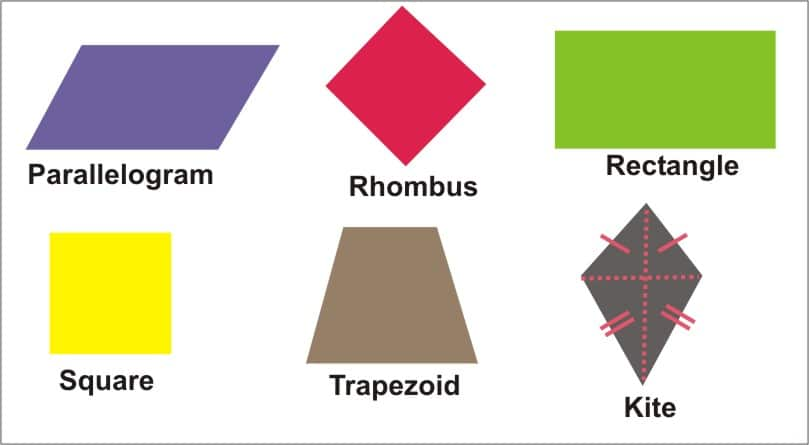Pentagon:

A plane figure with five straight angles and five straight sides.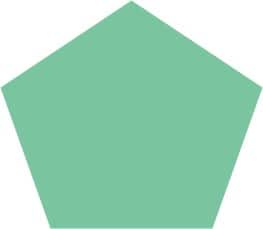Hexagon:

A plane figure with six straight angles and six sides.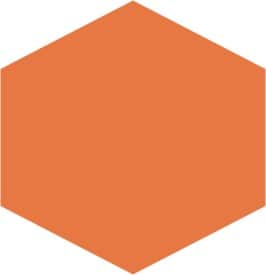Trigonometry:

A branch of mathematics that studies relationships involving lengths and angles of triangles is called trigonometry.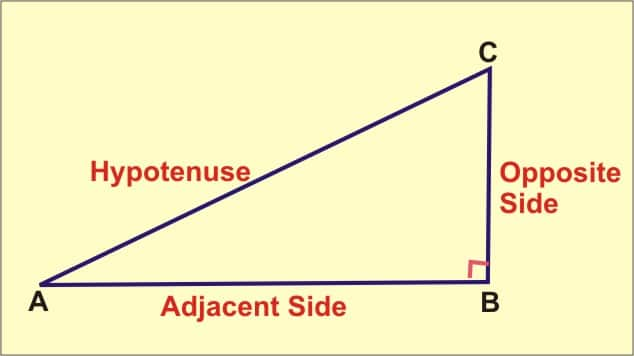The Adjacent side next to the angle is θ.
The hypotenuse is the longest side and the opposite side is opposite the θ.
There are six trig ratios: sine (sin), cosine (cos), tangent (tan), cosecant (csc), secant (sec), and cotangent (cot)

Formulas of the six trigonometric ratios:

Sin= Opp / Hyp
Cos= Adj / Hyp
Cosec= 1/Sin= Hyp / Opp
Sec = 1/cos= Hyp / Adj
Cot= 1/tan= Adj / Opp.

There is a simple trick to remember formula of first three trigonometric ratios:
Just remember “oSh aCh oTa” (oSh means for sine ratio formula is Opp/Hyp)
For example:
Finding all the 6 ratios for angle A.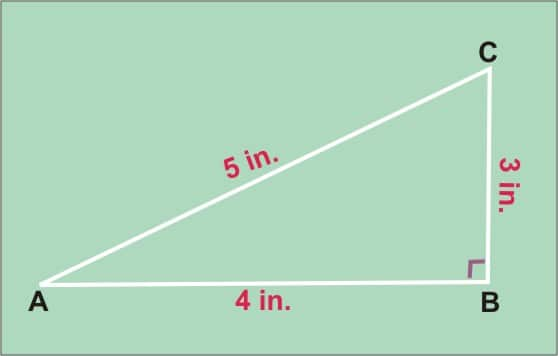Sin A= opp/hyp= 3/5
Cos A = adj/hyp= 4/5
Tan A= opp/adj =3/4
Cosec A = 1/sin= 5/3
Sec A = 1/cos= 5/4
Cot A = 1/tan=4/3
Likewise, finding all the ratios for angle B:
Sin B= 4/5
Cos B= 3/5
Tan B= 4/3
Cosec B= 5/4
Sec B= 5/3
Cot B= 3/4